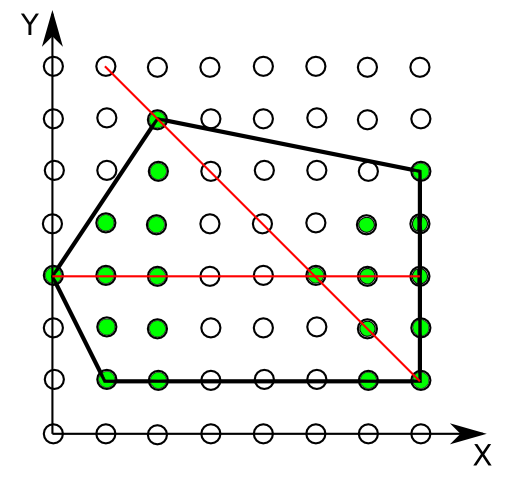### Problem Statement

You are given a convex polygon and you must count the number of N-cool points. A point is N-cool if it is a lattice point covered by the polygon and also an endpoint of some N-cool segment. A line segment is N-cool if it contains at least N lattice points that are covered by the polygon. A point is considered covered by the polygon if it is inside or on the boundary of the polygon. Endpoints of a segment are considered to be inside of it. A lattice point is a point with integer coordinates.

Consider the example in the picture below. Here N is equal to 6, and there are 21 6-cool points, marked green. Also two 6-cool segments, which are colored red, are shown.You are given int[]s x and y describing the vertices of the polygon (in no particular order). The coordinates of each vertex are specified by corresponding elements of x and y. Return the number of N-cool points.

### Definition

 Class: NCool Method: nCoolPoints Parameters: int[], int[], int Returns: int Method signature: int nCoolPoints(int[] x, int[] y, int n) (be sure your method is public)

### Notes

-A polygon is convex if it contains all the line segments connecting any pair of its points.

### Constraints

-x and y will each contain between 3 and 50 elements, inclusive.
-x and y will contain the same number of elements.
-All elements of x and y will be between 0 and 10000, inclusive.
-The number of lattice points inside or on the boundary of the specified polygon will not be greater than 500000.
-N will be between 2 and 500000, inclusive.
-The polygon specified by x and y will be convex and will have nonzero area.

### Examples

0)

 `{0, 1, 2, 7, 7}` `{3, 1, 6, 1, 5}` `6`
`Returns: 21`
 This is the example from the problem statement.
1)

 `{0, 1, 0}` `{0, 0, 1}` `2`
`Returns: 3`
 These three points form a triangle, whose sides are 2-cool segments, so all three vertices are 2-cool.
2)

 `{0, 0, 1, 2, 2, 1, 0, 0, 2}` `{0, 1, 2, 2, 1, 0, 0, 0, 2}` `3`
`Returns: 6`
 One point can be mentioned in the input two or more times.
3)

 `{0, 1, 1, 2, 2, 3, 3, 4, 4, 5}` `{1, 0, 2, 0, 2, 0, 2, 0, 2, 1}` `5`
`Returns: 4`
 There can be 3 points of the polygon, lying on the same line.
4)

 `{0, 1, 1, 2, 2, 3, 3, 4, 4, 5}` `{1, 0, 2, 0, 2, 0, 2, 0, 2, 1}` `4`
`Returns: 10`

#### Problem url:

http://www.topcoder.com/stat?c=problem_statement&pm=8320

#### Problem stats url:

http://www.topcoder.com/tc?module=ProblemDetail&rd=12173&pm=8320

griffon

#### Testers:

PabloGilberto , Olexiy , gawry

#### Problem categories:

Geometry, Search, Simple Math